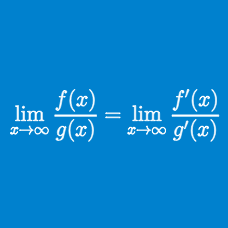Calculus

# L'Hopital's Rule - Convergence of Series

Consider the sequence $\left \{ a_n \right \}_{n \in \mathbb{N}}$ defined by \begin{aligned} a_1 &= \frac{3}{4} \\ a_n &= \frac{3^n}{4n} - \frac{3^{n-1}}{4 (n-1)}, n \in \mathbb{N} , n > 1 .\end{aligned} What is the value of $\displaystyle { \sum_{n=1}^{\infty} a_n } ?$

Consider the sequence $\left \{ a_n \right \}_{n \in \mathbb{N}}$ defined by \begin{aligned} a_1 &= 3 \cot 5 \\ a_n &= \frac{3}{n} \cot \frac{5}{n} -\frac{3}{n-1} \cot \frac{5}{n-1} , n \in \mathbb{N} , n > 1 .\end{aligned} What is the value of $\displaystyle { \sum_{n=1}^{\infty} a_n } ?$

Consider the sequence $\left \{ a_n \right \}_{n \in \mathbb{N}}$ defined by \begin{aligned} a_1 &= -\frac{2}{\log \cos 7} \\ a_n &= \frac{2}{(n-1)^2 \log \cos \frac{7}{n-1}}-\frac{2}{n^2 \log \cos \frac{7}{n}} , n \in \mathbb{N} , n > 1 .\end{aligned} What is the value of $\displaystyle { \sum_{n=1}^{\infty} a_n } ?$

Consider the sequence $\left \{ a_n \right \}_{n \in \mathbb{N}}$ defined by \begin{aligned} a_1 &= \frac{\log 3}{ \log 6} \\ a_n &= \frac{ \log (3n)}{\log (n+5)} - \frac{\log (3(n-1))}{\log ((n-1)+ 5)}, n \in \mathbb{N} , n > 1 .\end{aligned} What is the value of $\displaystyle { \sum_{n=1}^{\infty} a_n } ?$

Consider the sequence $\left \{ a_n \right \}_{n \in \mathbb{N}}$ defined by \begin{aligned} a_1 &= 27 \\ a_n &= {(3n)}^{\frac{3}{n}}- {(3(n-1))}^{\frac{3}{n-1}}, n \in \mathbb{N} , n > 1 .\end{aligned} What is the value of $\displaystyle { \sum_{n=1}^{\infty} a_n } ?$

×